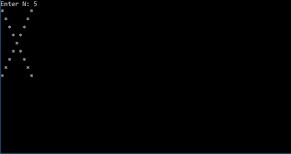# X Star Pattern C Program 3 Simple Ways | C Star Patterns

C Program to print the X star pattern – In this article, we will learn the multiple methods of how to print the X star pattern in C programming.

Suitable examples and sample programs have also been added so that you can understand the whole thing very clearly. The compiler has also been added with which you can execute it yourself.

The methods discussed in this piece are as follows:

• Using For Loop
• Using While Loop
• Using Do-While Loop

An X star pattern is a pattern that you have to print using the “*” symbol in the form of an X. This is what it looks like:In this case, the value of “n” has been set to 5. So, each arm of the X star pattern will have 5 “*” symbols.

Thus, the various methods used to print the X star pattern in C programming are as follows:

## Using For Loop

1) Read and store the entered number of rows and entered a symbol into the variables n, ch.

2) k is defined as k=n*2-1.

3) The outer for loop iterates through rows with the structure for(i=1;i<=k;i++).

4) The inner for loop iterates through columns with the structure for(j=1;j<=k;j++).

a) if j=i or j=k-i+1 condition is true then this loop prints symbol.

b) If the condition is false then this loop prints space.

5) Cursor comes to next line for each iteration of outer for loop.

Output:

### Using While Loop

1) k=n*2-1.

2) i=1, The outer while loop with the condition i<=k iterates through rows until the condition becomes false.

3) j=1, The inner while loop iterates through columns until the condition j<=k becomes false.

a) Prints symbol if j=i or j=k-i+1 the condition is true. Otherwise, it prints space.

b) j value increased by 1.

4)  The cursor comes to next line. i value increased by 1 of the outer while loop.

Output:

#### Using Do-While Loop

1) k=n*2-1.

2) i=1, The outer do-while loop iterates through rows.

3) j=1, The inner do-while loop iterates through columns.

a) if j=i or j=k-i+1 the condition is true then loop prints symbol.

b) otherwise, it prints space.

c) j value increased by 1.

Checks the condition j<=k.If the condition is true then inner loop iterates again.Repeats until the condition becomes false.

4) The cursor comes to next line.

5) i value increased by 1.Checks the condition i<=k.if the condition is true then outer loop iterates again. Repeats until the condition becomes false.

Output:
x

## C Program To Check If Alphabet, Digit or Special Character | C Programs

C program to check for an alphabet, digit or special character – In this article, ...